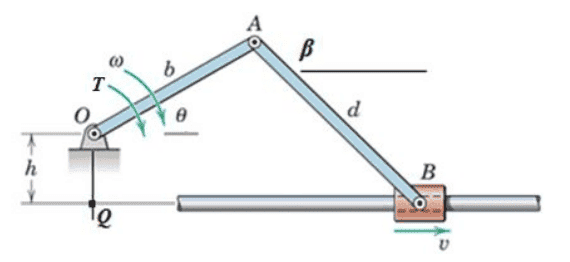# Acceleration of Center of Mass

Thread moved from the technical forums, so no Homework Template is shown
I have a model of two slender rods (call them OA and AB) connected with a pin at A, anchored at O with a pin, and attached to horizontal slider at B. I am supposed to show graphs of different variables in Matlab as the angle theta changes. One of these steps is to show the acceleration of the center of mass of link OA. It is given that link OA has a constant angular velocity 'omega' in the CW direction. Dumb question, but if the rod has constant angular velocity, would a point half way down this link (center of mass) also have zero acceleration? (Note, I am asked to find linear acceleration, i.e. a=alpha/r)

•Lakshit

Khashishi
Can you draw a diagram or something? It's not clear what theta is, and what a horizontal slider is, and what alpha is.

•Lakshit
Apologies, here is an image of the problem.This is the same image as what is used in my problem, except that T direction (torque I assume) is placed on this diagram.

Khashishi